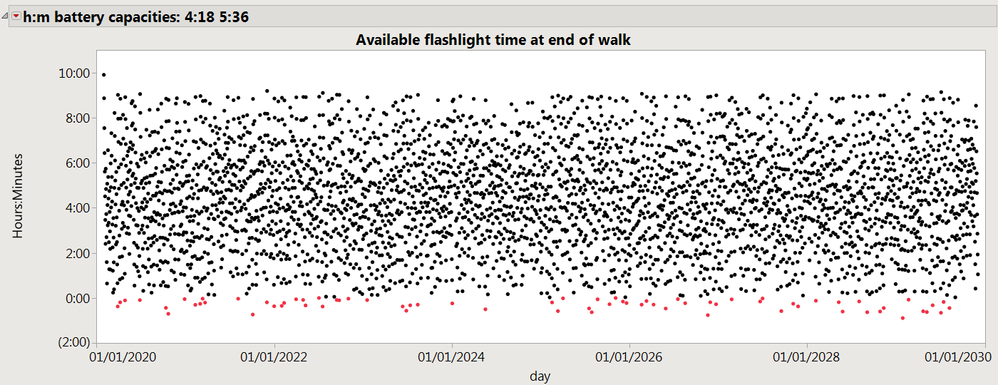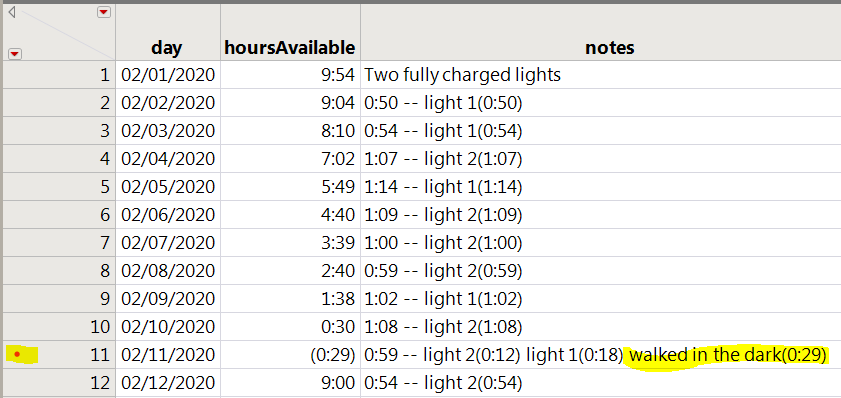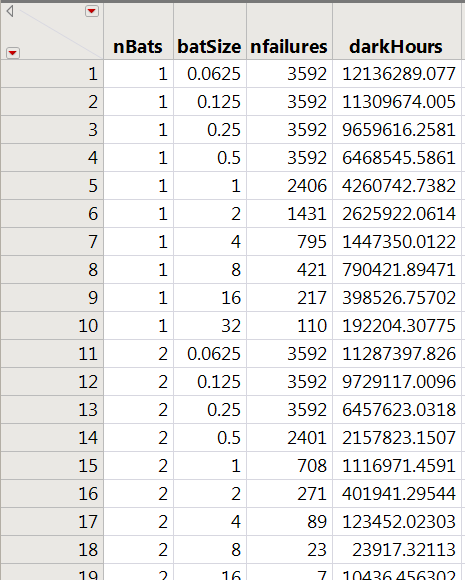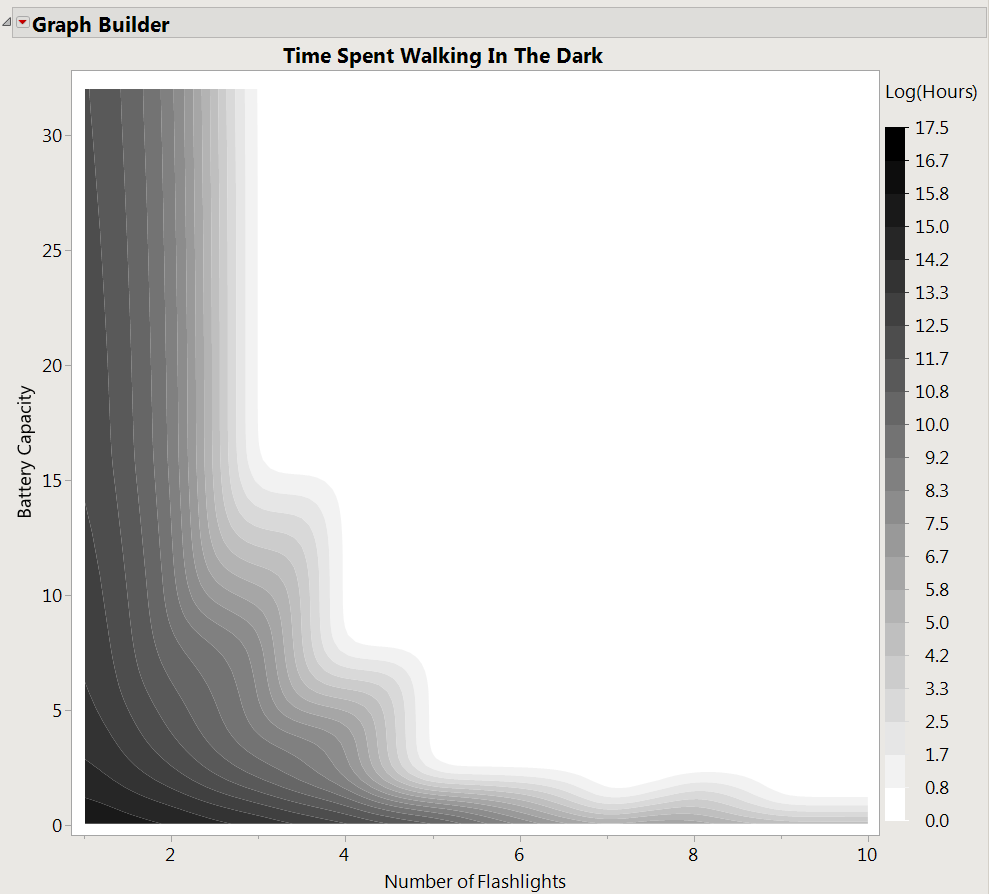Choose Language Hide Translation Bar
Battery usage

I carry two flashlights when I walk before sunrise; each has about a 5 hour battery and the walk lasts about an hour. I try to use the same flashlight until it runs down, and about every fifth day I get to switch lights during the walk. The old light goes on charge, and I try to remember which one to use the next day. This plan actually works pretty well, even if I forget to switch lights the next day.

But what would happen if I randomly chose a flashlight each day? How often would I expect to have two 15-minute lights, and no light for the second half of the walk? The attached JSL is a simulation that tries to answer the question; first let's see the answer:The red dots below 0 show where the two flashlights both failed, and how badly

The title bar says this simulation was for two lights, one with a 4+ hour battery and the other with a 5+ hour battery. Most days there are several hours left in the pair of lights. The JSL begins with a user function, maketable, because the code will be reused below to make another graph.

``````maketable = function({capacity},// an array with the battery capacities expressed as time

The first set of { curly braces } holds the parameter to the function, capacity, which will be a small matrix with the hour-capacity of each flashlight. The second set holds the local variables for the function; if the JSL that calls the function happens to use the same variable names, the caller's variables won't be changed by this function.

``````	charge = capacity; // charge[] tracks the remaining charge

// expression that computes random on time for flashlight
ontime = Expr(
As Date( In Hours( Min( 2, Max( .5, Random Normal( 1, .1 ) ) ) ) ) // usually about 1-hour walk
);

// table to collect results
dt = New Table( "Light Schedule",
New Column( "day", Numeric, "Ordinal", Format( "m/d/y", 10 ), Input Format( "m/d/y" ), Set Values( {1feb2020} ) ),
New Column( "hoursAvailable", Numeric, "Continuous", Format( "hr:m", 12 ), Set Values( {sum(charge)} ) ),
New Column( "notes", Character, Set Values( {"Two fully charged lights"} ), Set Display Width( 500 ) ),
Set Label Columns( :notes )
);``````

Above, the batteries become initially fully charged by copying capacity into charge. Then an expression is assigned to ontime, which represents the distribution of my walk times...an hour, more or less. And finally, dt is set to a new data table that this function will eventually return to the caller. The table includes a day counter column, a column that shows how many hours were available in the flashlights after the walk, and a column with computer-generated notes about what happened.

``````	// run the simulation for about 10 years
For( today = 2feb2020, today <= 2dec2029, today += In Days( 1 ),
remainingWalkTime = ontime; // gets a new random duration for the length of an early morning walk
message = Format( remainingWalkTime, "hr:m" ) || " --"; // total walk duration
While( remainingWalkTime > 0 & Sum( charge ) > 0, // also give up when there are no charged lights remaining
// choosing randomly might be too slow if there are many uncharged lights, but there are only 2 or 3...
light = Random Integer( N Rows( capacity ) ); // choose from available lights
If( charge[light] > 0, // if it is a charged light, use it
usage = Min( remainingWalkTime, charge[light] ); // only take what is needed, up to available charge
remainingWalkTime -= usage;
charge[light] -= usage;
message ||= " light " || Char( light ) || "(" || Format( usage, "hr:m" ) || ")";
);
);``````

The first part of the simulation loop, above, loops through the days from 2020 to 2030. (The dates are not really used except to label the graph; the duration is roughly 10*365 days...you'll see about 3500 rows in the table later.) remainingWalkingTime is set to about an hour and becomes the initial value for message, which will have some more text that becomes the notes for the day. Inside the For loop, there is a While loop that randomly chooses a flashlight for part of the walk. The For loop continues to choose flashlights until the walk is over or there are no flashlights with remaining charge. The chosen light is either used up, or used enough to finish the walk. Then the message is updated to say which light was used, and for how long.

``````		// update data table
If( remainingWalkTime > 0,
Row State() = Color State( "red" );
// the next line makes the charge go below zero on the first battery;
// that makes column 1 have negative numbers, and makes the graph do
// a better job of visualizing how long the walk in the dark lasted.
message ||= " walked in the dark(" || Format( remainingWalkTime, "hr:m" ) || ")";
charge -= remainingWalkTime;
remainingWalkTime = 0; // maybe the sun is coming up
);
dt:day = today;
dt:hoursAvailable = sum(charge);
dt:notes = message;
deadBatteries = Loc( charge <= 0 );
message ||= " recharged" || Char( deadBatteries );
);``````

The last part of the simulation, above, adds a row to the table to represent today, colors it red if there was remainingWalkingTime left over, and sets the day, hoursAvailable, and notes for the new row. Finally, the batteries are recharged; the loc function finds the indexes, or locations, in the charge matrix that went below zero. Then, for those locations only, the charge value is reset to the capacity value.

``````	dt; // return value
);``````

And that (above) ends the function; the last statement's value is returned to the caller. dt, all by itself, is a really simple statement that evaluates to ... dt. You can also use the return function: return(dt); anywhere in the function.

``````// the following line determines how many flashlights are in the simulation
capacity = matrix({In Hours( 4.3 ),In Hours( 5.6 )}); // one battery is much older, less capacity.

dt = maketable(capacity);

// graph the results
dt << Graph Builder(
Size( 1388, 500 ),
Show Control Panel( 0 ),
Show Legend( 0 ),
Variables( X( :day ), Y( :hoursAvailable ) ),
Elements( Points( X, Y, Legend( 9 ) ) ),
SendToReport(
Dispatch( {}, "Graph Builder", OutlineBox, {Set Title( title="h:m battery capacities: "; for(icap=1,icap<=nRows(capacity),icap+=1,title||=format(capacity[icap],"hr:m")||" ";); title )} ),
Dispatch({},"day",ScaleBox,	{Format( "m/d/y", 10 ), Min( 3660681600 ), Max( 3976300800 ), Interval( "Year" ), Inc( 2 ), Minor Ticks( 0 )}),
Dispatch( {}, "graph title", TextEditBox, {Set Text( "Available flashlight time at end of walk" )} ),
Dispatch( {}, "Y title", TextEditBox, {Set Text( "Hours:Minutes" )} ),
Dispatch( {}, "400", LegendBox, {Set Title( "" )} )
)
);``````

Finally, above, call maketable with a capacity that defines two batteries. The dt on the left of the = is a global variable, assigned the result from the function on the right of the =. The dt in the function is in the local list at the beginning of the function, and does not change the global dt. Once the data table is created, graph builder can make the graph (way up above.) Don't write a graph builder script from scratch! Use the gui to get what you want, then save the script. I edited this one to add the custom outline title with the battery capacities.

The table that graph builder (above) used looks like this:Output from maketable() function showing (negative) hours and notes

Since maketable() takes an argument for the capacity of the batteries, I can run it multiple times. I don't really plan to carry additional flashlights, but I do want to know how many flashlights, of what capacity, best solve the problem. Here's some JSL that calls maketable from a loop, and collects another table of answers from the tables maketable returns:

``````// make a table-of-tables; use from 1 to 10 flashlights with batteries from 1/16 to 32 hours
dtreport = New Table( "lights",
New Column( "nBats", Numeric, "Continuous", Format( "Best", 12 ), Set Values( [] ) ),
New Column( "batSize", Numeric, "Continuous", Format( "Best", 12 ), Set Values( [] ) ),
New Column( "nfailures", Numeric, "Continuous", Format( "Best", 12 ), Set Values( [] ) ),
New Column( "darkHours", Numeric, "Continuous", Format( "Best", 12 ), Set Values( [] ) )
);``````

dtreport is the data table that will hold the aggregated answers.

``````For( nLights = 1, nLights <= 10, nLights += 1,
For( batLife = 1 / 16, batLife <= 32, batLife *= 2,
dt = maketable( Repeat( inhours(batLife), nLights ) );``````

Above, a pair of loops will run through 100 combinations, making a new dt each time.

``````		// count the failures and duration of failures
remaining = dt[0, "hoursAvailable"];
failures = Loc( remaining < 0 );
nfail = N Rows( failures );
darkSum = As Date( -Sum( remaining[failures] ) );
dtreport:nBats[nrows(dtreport)]=nLights;
dtreport:batSize[nrows(dtreport)]=batLife;
dtreport:nfailures[nrows(dtreport)]=nfail;
dtreport:darkHours[nrows(dtreport)]=darkSum;``````

Above, remaining grabs the hoursAvailable column as a matrix so I can use loc() again to locate the failures. I tried the number of failures and the total time of the failures and got similar results in the graph. Add a row to the dtreport table and fill it in.

``````		Close( dt, "nosave" );
)
);``````

Close the dt tables in the loop when no longer needed so there won't be 100 windows open.

Now the table just created looks like this:.0625 hour will probably never be enough, even with 10 flashlights

I can see that more lights and better batteries helps. The JSL (saved from graph builder's gui) looks like this

``````dtreport<<Graph Builder(
Size( 868, 854 ),
Show Control Panel( 0 ),
Variables( X( :nBats ), Y( :batSize ), Color( Transform Column( "Log[darkHours+1]", Formula( Log( :darkHours + 1 ) ) ) ) ),
Elements( Contour( X, Y, Legend( 11 ), Smoothness( 0.0775532495758591 ), Number of Levels( 21 ) ) ),
SendToReport(
Dispatch( {}, "nBats", ScaleBox, {Min( 0.847222222222222 ), Max( 10.1282968574635 ), Inc( 2 ), Minor Ticks( 1 )} ),
Dispatch( {}, "batSize", ScaleBox, {Min( -0.435203094777562 ), Max( 32.806718570536 ), Inc( 5 ), Minor Ticks( 0 )} ),
Dispatch(
{},
"400",
ScaleBox,
{Legend Model(
11,
Properties(
0,
{gradient( {Color Theme( {"White to Black Copy", 2051, {"Black", "White"}} ), Width( 12 ), Reverse Gradient( 1 )} )},
Item ID( "Log[darkHours+1]", 1 )
)
)}
),
Dispatch( {}, "graph title", TextEditBox, {Set Text( "Time Spent Walking In The Dark" )} ),
Dispatch( {}, "X title", TextEditBox, {Set Text( "Number of Flashlights" )} ),
Dispatch( {}, "Y title", TextEditBox, {Set Text( "Battery Capacity" )} ),
Dispatch( {}, "400", LegendBox, {Set Title( "Log(Hours)" )} )
)
);``````

And the resulting graph:More flashlights or more batteries keeps me out of the dark

The contour plot shows the results of the 100 simulations; it appears that three flashlights with 17 hour capacities, or four with 8 hour capacities might do the trick. But with only two flashlights, choosing a light a random, and only charging when dead, there might not be a capacity that would keep the lights on.

Article Labels
Article Tags